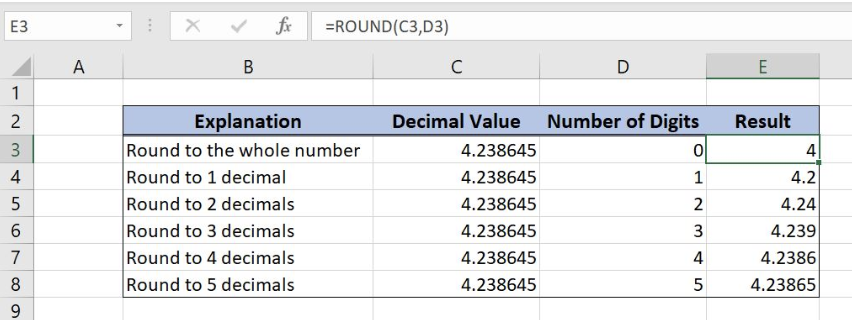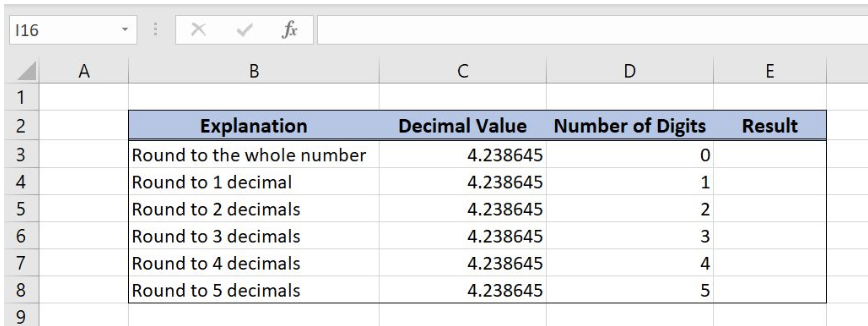Get instant live expert help with Excel or Google Sheets“My Excelchat expert helped me in less than 20 minutes, saving me what would have been 5 hours of work!”

#### Post your problem and you’ll get Expert help in seconds.

Your message must be at least 40 characters
Our professional Expert are available now. Your privacy is guaranteed.

# How to Round Decimal Values in Excel

Whenever we are working with decimal values in Excel, we need to format them appropriately. One of the best ways to format decimal numbers is using the ROUND function. In this tutorial, we will learn how to the particular decimal value that we choose.Figure 1. Final result

## Syntax of the ROUND formula

`=ROUND(number, num_digits)`

The parameters of the ROUND function are:

• number – a decimal number which we want to round
• num_digits – a number of decimal places to which we want to round a number

## Setting up Our Data for the ROUND Function

Our table consists of 4 columns: “Explanation” (column B), “Decimal Value” (column C), “Number of Digits” (column D) and “Result” (Column E). In column “Result” we want to get a rounded number, based on “Decimal Value” and “Number of Digits” columns.Figure 2. Data that we will use in the ROUND example

## Round Decimal Values Using the ROUND Function

We want to round the value from cell C3 by using the number of digits from cell D3l. The result will be stored in E3.

Formula:

`=ROUND(C3, D3)`

The parameter number is the cell C3 (4.238645), while the parameter num_digits is the cell D3 (0).

To apply the SUMIFS function, we need to follow these steps:

• Select cell E3 and click on it
• Insert the formula: =ROUND(C3,D3)
• Press enter
• Drag the formula down to the other cells in the column by clicking and dragging the little “+” icon at the bottom-right of the cell.Figure 3. Using the ROUND function to round decimal values

As a result, we get value 4 in the cell E3. Putting 0 as num_digits means that we want to round number to the nearest whole number, which is 4. We must know that the function rounds number 1-4 down and 5-9 up. Because of that, 4.238645 rounding to 1 decimal is 4.2, while rounding to 2 decimals is 4.24

Most of the time, the problem you will need to solve will be more complex than a simple application of a formula or function. If you want to save hours of research and frustration, try our live Excelchat service! Our Excel Experts are available 24/7 to answer any Excel question you may have. We guarantee a connection within 30 seconds and a customized solution within 20 minutes.

### Did this post not answer your question? Get a solution from connecting with the expert.Another blog reader asked this question today on Excelchat:
Solution examplesI am trying to utilize a complicated sumif or sumifs formula that can pull a total amount from a date and a drop down box from another tab.
Solved by E. B. in 15 minsI have a spreadsheet that totals invoices. A bunch of cells at the bottom of the spreadsheet calculates totals based on which department the invoice is charged to. I'd like to add a function where it also considers the date (so if it's an invoice in January, it goes to a January total, February, etc.). But I can't figure out how to write it properly...
Solved by K. J. in 27 minsIf I want to write a formula that states" "If this name shows up K24, and this word shows up in J24 pull this number from M24" I can creat that formula - however I need to drag this down so it pulls from row 24 - 60 - is there way to do this?
Solved by F. D. in 40 minsamending a formula so that there is another criteria and if it does not meet this criteria then we stick with the original criteria
Solved by A. B. in 40 minsSo im trying to use a sumif formula, but i need it to not look for a range for one specific criteria but instead look for what one specific field says. Example Below =sum(sumifs(QuantityMC,TeamsMC,A6,TypeMC,B2)) The above formula works as on the specific MC sheet an entire column is dedicated for "TypeMC". I want to be able to delete this column and have "TypeMC" to be a single field as it will be the same for every item on that sheet but may differ on a different sheet.
Solved by M. H. in 45 mins## Subscribe to Excelchat.coAnother blog reader asked this question today on Excelchat: